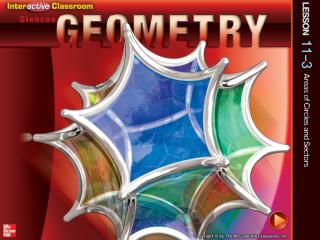DownloadDownload PresentationSplash Screen

# Splash Screen

Download Presentation## Splash Screen

- - - - - - - - - - - - - - - - - - - - - - - - - - - E N D - - - - - - - - - - - - - - - - - - - - - - - - - - -
##### Presentation Transcript

1. Splash Screen

2. Five-Minute Check (over Lesson 11–2) CCSS Then/Now New Vocabulary Key Concept: Area of a Circle Example 1: Real-World Example: Area of a Circle Example 2: Use the Area of a Circle to Find a Missing Measure Key Concept: Area of a Sector Example 3: Real-World Example: Area of a Sector Lesson Menu

3. Find the area of the figure. Round to the nearest tenth if necessary. A. 202 units2 B. 198 units2 C. 62.7 units2 D. 28.4 units2 5-Minute Check 1

4. Find the area of the figure. Round to the nearest tenth if necessary. A. 96 units2 B. 92.4 units2 C. 83.1 units2 D. 81.8 units2 5-Minute Check 2

5. Find the area of the figure. Round to the nearest tenth if necessary. A. 70 units2 B. 72.5 units2 C. 75 units2 D. 77.5 units2 5-Minute Check 3

6. Find the area of the figure. Round to the nearest tenth if necessary. A. 58.5 units2 B. 117 units2 C. 198 units2 D. 234 units2 5-Minute Check 4

7. Trapezoid LMNO has an area of 55 square units. Find the height. A. 6 units B. 5 units C. 4 units D. 3 units 5-Minute Check 5

8. The area of a kite is 120 square meters. The length of one diagonal is 15 meters. Find the length of the other diagonal. A. 4 m B. 8 m C. 16 m D. 1800 m 5-Minute Check 6

9. Content Standards G.C.5 Derive using similarity the fact that the length of the arc intercepted by an angle is proportional to the radius, and define the radian measure of the angle as the constant of proportionality; derive the formula for the area of a sector. G.GMD.1 Give an informal argument for the formulas for the circumference of a circle, area of a circle, volume of a cylinder, pyramid, and cone. Mathematical Practices 1 Make sense of problems and persevere in solving them. 6 Attend to precision CCSS

10. You found the circumference of a circle. • Find areas of circles. • Find areas of sectors of circles. Then/Now

11. sector of a circle • segment of a circle Vocabulary

12. Concept 1

13. Area of a Circle MANUFACTURING An outdoor accessories company manufactures circular covers for outdoor umbrellas. If the cover is 8 inches longer than the umbrella on each side, find the area of the cover in square inches. The diameter of the umbrella is 72 inches, and the cover must extend 8 inches in each direction. So, the diameter of the cover is 8 + 72 + 8 or 88 inches. Divide by 2 to find that the radius is 44 inches. Example 1

14. Area of a Circle Area of a circle Substitution Use a calculator. Answer: The area of the cover is about 6082 square inches. Example 1

15. A swimming pool company manufactures circular covers for above ground pools. If the cover is 1 foot longer than the pool on each side, find the area of the cover. A. 62.8 ft2 B. 254.5 ft2 C. 314.2 ft2 D. 1256.6 ft2 Example 1

16. Take the positive square root of each side. = r Use the Area of a Circle to Find a Missing Measure ALGEBRAFind the radius of a circle with an area of 58 square inches. Area of a circle Substitution Divide each side by . 4.3 ≈ r Simplify. Answer: The radius of the circle is about 4.3 in. Example 2

17. ALGEBRA Find the radius of a circle with an area of 45 square inches. A. 3.8 in. B. 4.5 in. C. 5.7 in. D. 7.6 in. Example 2

18. Concept 2

19. Area of a Sector PIEA pie has a diameter of 9 inches and is cut into 10 congruent slices. What is the area of one slice to the nearest hundredth? Step 1 Find the arc measure of a pie slice. Since the pie is equally divided into 10 slices, each slice will have an arc measure of 360 ÷ 10 or 36. Step 2 Find the radius of the pie. Use this measure to find the area of the sector, or slice. The diameter is 9 inches, so the radius is 4.5 inches. Example 3

20. Area of a Sector Area of a sector x = 36 and r = 4.5 Use a calculator. Answer: The area of one slice of pie is about6.36 square inches. Example 3

21. PIZZAA pizza has a diameter of 14 inches and is cut into 8 congruent slices. What is the area of one slice to the nearest hundredth? A. 16.21 in2 B. 19.24 in2 C. 26.43 in2 D. 38.48 in2 Example 3

22. End of the Lesson